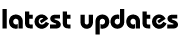Physics : Electric Current

In the late 1790s, Count Alessandro Volta made the first battery. The first practical primary cell (non-rechargeable) was produced by John Frederic Daniell (1790-1845) in 1836, using zinc and copper electrodes. This was followed by the first secondary (rechargeable) cell invented by Gaston Plante (1834-89) in 1859. This cell, based upon the use of lead and sulfuric acid, is still employed as the car battery. The Leclanche ‘Dry’ Cell of the 1860s was developed into the modern ordinary torch battery. It has a zinc case and a carbon electrode down its centre.

In 1827, George Simon Ohm introduced the concept of resistance (to the flow of an electric current) and stated Ohm’s Law: I = V/R which translates as: I, the current in amperes (amps) in the conductor, V, the potential difference in volts across the ends of the conductor and R, the resistance of the conductor.

In 1831, Michael Faraday confirmed his hypothesis that magnetism could produce electricity when he found that a magnet moving in a coil of wire induced a current in the wire when the wire was present in an electric circuit. These discoveries soon led to the electric motor and to the dynamo, a device for generating electricity by spinning a coil of wire between the poles of a suitably shaped magnet.

James Clerk Maxwell predicted in 1873 that by using oscillating electric currents it should be possible to generate electromagnetic waves which travel at the speed of light. In the late 1880s, Heinrich Hertz produced such waves, which were soon used for wireless transmissions.

Measuring current

1 amp current has 6 x 1023/96,500 or approximately 6 x 1018 electrons passing a given point in the circuit every second. A one-volt battery supplies one joule of energy to eachcoulomb of electric charge that it produces. Mechanical power is measured in watts (joules per second). If a 6v battery is supplying 5 amps (5 coulombs per second), it is thus producing 6 joules per coulomb and a total of 5 x 6 = 30 joules per second. The general formula for power supplied is: P = V x I where P is the power in watts, V is the voltage (also known as Potential Difference) and I is the current in amps.My Great Web page